# KVPY-SA 2019 Chemistry Paper with Solutions

KVPY-SA 2019 Chemistry paper with solutions is provided on this page. The question paper with solutions is a great study resource for aspirants who are preparing to write the exam. The KVPY paper will enable them to understand the questions asked in the paper and learn the different methods to solve them and also get a deeper understanding of the concepts. Candidates can download the KVPY SA 2019 question paper below. Kishore Vaigyanik Protsahan Yojana also known as KVPY is a scholarship program conducted annually by the Department of Technology & Science, Government of India. KVPY examination aims at encouraging the students to pursue a research career in basic sciences. Scholarships and Contingency are granted to the candidates qualifying the talent test (up to the pre PhD level). KVPY- SA is an examination conducted for students who have scored 75% aggregate marks in Maths and Science subjects in their Class 10 board exam and have enrolled in Class 11 Science stream.

### KVPY SA 2019 - Chemistry

Question 1: The hybridizations of N, C and O shown in the following compound respectively, are –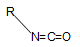1. a. sp2, sp, sp2
2. b. sp2, sp2, sp2
3. c. sp2, sp, sp
4. d. sp, sp, sp2

Solution:

Hybridisation of

O = 1 B.P. (σ bond) + 2 l.p. = 3 ⇒sp2

C = 2 B.P (σ bond) = 2 ⇒ sp

N = 2 B.P. (σ bond) + 1l.P. = 3 ⇒ sp2

So, option (A) is correct

Question 2: The following compounds are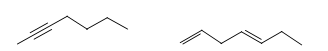1. a. geometrical isomers
2. b. positional isomers
3. c. optical isomers HCl
4. d. functional group isomers

Solution:

a. CH3 – C ≡ C – CH2 – CH2 – CH2 – CH3 ⇒ C7H12 {– yne compound}

b. CH2 = CH – CH2 – CH = CH – CH2 – CH3⇒ C7H12 {– diene compound}

Both –yne and –diene compounds are having same molecular formula but different functional group. So, they are functional group isomers of each other.

Question 3: The major product of the following reaction is -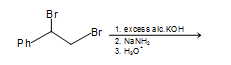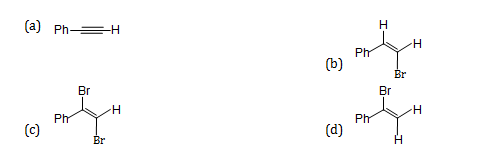Solution: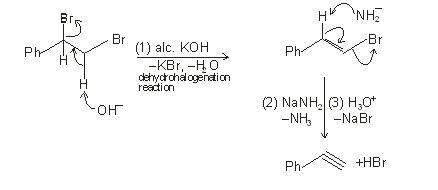Question 4: IUPAC name of the following compound is –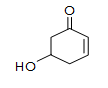1. a. 1-hydroxycyclohex-4-en-3-one
2. b. 1-hydroxycyclohex-3-en-5-one
3. c. 3-hydroxycyclohex-5-en-1-one
4. d. 5-hydroxycyclohex-2-en-1-one

Solution: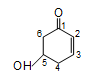OH → Hydroxy (prefix)

= (double bond) →en (primary suffix)

Ketone →one (secondary suffix)

Root word →cyclo hex

So, IUPAC name: 5–hydroxy cyclohex–2–en–1–one

Question 5: In water-gas shift reaction, hydrogen gas is produced from the reaction of steam with -

1. a. Methane
2. b. Coke
3. c. Carbon Monoxide
4. d. Carbon Dioxide

Solution:

The water-gas shift reaction is an equilibrium process. In which carbon monoxide and steam converting into hydrogen and carbon dioxide.

CO + H2O → CO2 + H2

(steam)

Question 6: Treatment with lime can remove the hardness of water caused by -

1. a. CaCl2
2. b. CaSO4
3. c. Ca(HCO3)2
4. d. CaCO3

Solution:

Lime (Ca(OH)2) is used to remove the temporary hardness of water causes by salts of Ca and Mg.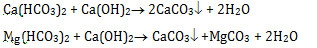Question 7: The most polarizable ion among the following is

1. a. F
2. b. I
3. c. Na+
4. d. Cl

Solution:

According to Fajan’s rule

Polarization ∝ size of anion ∝ 1/size of cation

So, out of F, I, Na+ ,Cl. I- anion has the largest in size. So, it’s most polarizable.

Question 8: For a multi-electron atom, the highest energy level among the following is -

1. a. n =5, l = 0, m =0, s = +1/2
2. b. n =4, l = 2, m =0, s = +1/2
3. c. n =4, l = 1, m =0, s = +1/2
4. d. n =5, l = 1, m =0, s = +1/2

Solution:

Option

(A) n = 5, l= 0, m = 0, s = + ½ ⇒ 5s orbital

(B) n = 4, l = 2, m = 0, s = + ½ ⇒ 4d orbital

(C) n = 4, l = 1, m = 0, s = + ½ ⇒ 4p orbital

(D) n = 5, l = 1, m = 0, s = + ½ ⇒ 5p orbital

So according to (n + l) rule, increasing order of energy level: higher the value of (n+l), higher will be the energy. If (n+l) value is same, then check the value of n. Higher the value of n, higher will be the energy.

4p< 5s < 4d < 5p

So, 5p is highest energy level.

Question 9: The oxide which is neither acidic nor basic is -

1. a. As2O3
2. b. Sb4O10
3. c. N2O
4. d. Na2O

Solution:

Some non-metal oxides do not show any acidic or basic nature. They are neutral oxide.

Example: H2O, CO, NO, N2O etc.

As2O3& Sb4O10 are amphoteric and Na2O is a basic oxide.

Question 10: The element whose salts cannot be detected by the flame test is -

1. a. Mg
2. b. Na
3. c. Cu
4. d. Sr

Solution:

Flame test:

(A) Mg → None of the salts gives [but for burning Mg metal (intense white)]

(B) Na → yellow.

(C)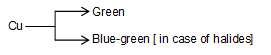(D) Sr → Crimson red to scarlet

Question 11: The plot of concentration of a reactant vs. time for a chemical reaction is shown below: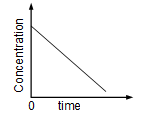The order of this reaction with respect to the reactant is

1. a. 0
2. b. 1
3. c. 2
4. d. not possible to determine from this plot

Solution:

Let, the reaction be zero order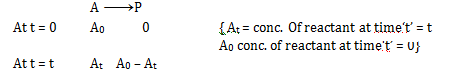So, according to law of mass action

R ∝ [A]0 at time =t

r=k [Since, [A]0=1] {k = rate constant}

We know, rate= Change in concentration/change in time

Therefore, -dA/dt =k ⇒ -dA = kdt

On integrating both the side and applying limits

$-\int_{A_{0}}^{A_{t}} dA=k \int_{0}^{A_{t}} dt \Rightarrow[A]_{0}^{A_{t}}=-k[t]_{0}^{t}$

At-A0 =-kt

At = -kt+A0

On comparing with straight line equation

y=mx+c

At = -kt+A0

So, plot of At vs t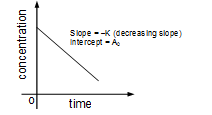So order of reaction with respect to reactant is zero order.

Question 12: During the free expansion of an ideal gas in an isolated chamber,

1. a. internal energy remains constant
2. b. internal energy decreases
3. c. work done on the system is negative
4. d. temperature increases

Solution:

Free expansion of an ideal gas in an isolated chamber is irreversible adiabatic free expansion process.

⇒ In an adiabatic process → Heat is constant

So, q = 0 (isolated chamber)

⇒ In irreversible process work done → w = – Pext. ΔV

So, w = 0

We know, Internal energy (ΔU) = q + w = 0

Hence, internal energy is constant.

Question 13: The number of moles of water present in a spherical water droplet of radius 1.0 cm is [Given the density of water in the droplet = 1.0 g cm–3]

1. a. π/18
2. b. 2π/27
3. c. 24 π
4. d. 2π/9

Solution:

Given:-

Radius of water droplet = 1.0 cm

Density of water droplet = 1.0 g/cm3

We know, density = mass/ volume ⇒ mass = volume

No. of moles = mass/molar mass {mass = volume}

So, moles = volume/molar mass {volume of sphere = (4/3) πr3 }

Mole = $\frac{\frac{4}{3}\pi (1.0)^{3}}{18}$ {molar mass of H2O = 18 g mol–1}

No. of moles = 2π/27

Question 14: Among the following, the correct statement about cathode ray discharge tube is

1. a. the electrical discharge can only be observed at high pressure and at low voltages
2. b. in the absence of external electrical or magnetic field, cathode rays travel in straight lines
3. c. the characteristics of cathode rays depend upon the material of electrodes
4. d.the characteristics of cathode rays depend upon the gas present in the cathode ray tube

Solution:

Cathode rays or stream of electrons move in a straight line without any deflection in the absence of electric and magnetic field. But in the presence of the electric field, the cathode rays are deflected towards the positive pole.

Question 15: For a spontaneous process

1. a. enthalpy change of the system must be negative
2. b. entropy change of the system must be positive
3. c. entropy change of the surrounding must be positive
4. d. entropy change of the system and surrounding (universe) must be positive

Solution:

For a spontaneous process ΔG < 0

So, we know, ΔG = ΔH – T ΔS

So, for spontaneous Reaction, ΔH = –ve, ΔS = +ve

Therefore, entropy change of the system and surrounding (universe) must be positive.

Question 16: PbO2 is obtained from

1. a. the reaction of PbO with HCl
2. b. thermal decomposition of Pb(NO3)2 at 200 °C
3. c. the reaction of Pb3O4 with HNO3
4. d. the reaction of Pb with air at room temperature

Solution: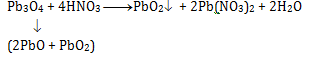Question 17: For one mole of a van der Waals gas, the compressibility factor $z(=\frac{PV}{RT})$ at a fixed volume will certainly decrease if

[Given: "a", "b" are the standard parameter for van der Waals gas]

1. a."b" increases and "a" decreases at a constant temperature
2. b. "b" decreases and "a" increases at a constant temperature
3. c. temperature increases at constant "a" and "b" values
4. d. "b" increases at constant "a" and temperature

Solution:

a → intermolecular force of attraction

b → volume occupied by molecules.

We know, compressibility factor Z = Vreal/Videal = PV/RT

To decreases Z value, Vreal (numerator) should decrease which decreases when ‘a’ increases & ‘b’ decreases at constant temperature

Question 18: The correct statements among the following

(i) E2s(H) > E2s (Li) > E2s (Na) > E2s (K)

(ii)The maximum number of electrons in the shell with principal quantum number n is equal to 2n2

(iii)Extra stability of half-filled subshell is due to smaller exchange energy.

(iv)Only two electrons, irrespective of their spin, may exist in the same orbital.

1. a. i and ii
2. b. ii and iii
3. c. iii and iv
4. d. i and iv

Solution:

(i) We know that, E = – 13.6 × (z2/n2)ev

So, the higher the value of Z (atomic number) lower is the value of E2s

(ii) Maximum number of electron in the shell = 2n2

(iii) The extra stability of half-filled subshell is due to higher exchange energy.

(iv) Only two electrons with opposite spin can exist in the same orbital. According to Pauli's exclusion principle. So, statement (i) and (ii) are correct.

Question 19: An organic compound contains 46.78% of a halogen X. When 2.00 g of this compound is heated with fuming HNO3 in the presence of AgNO3, 2.21 g AgX was formed. The halogen X is

[Given: atomic weight of Ag = 108, F = 19, Cl = 35.5, Br = 80, I = 127]

1. a. F
2. b. Cl
3. c. Br
4. d. I

Solution:

Given: % if X in organic compound = 46.78%

Weight of organic compound = 2.00g

Weight of AgX formed = 2.21 g

So, weight of X in organic compound =2 x (46.78/100) = 0.94 gm

Let, the molecular weight of X = xg mol–1

So, by the law of equivalence

No. of eq. of X = no. of eq. of AgX

Mole × valency factor = mole × valency factor

$\frac{0.94}{x}\times 1=\frac{2.21}{108+x}\times 1$

(101.52 + 0.94x) =2.21x

101.52 = 1.27x

x = 79.9 ≈ 80

So, halogen X is Bromine (Br)

Question 20: An organic compound X with molecular formula C6H10, when treated with HBr, forms a gem dibromide. The compound X upon warming with HgSO4 and dil. H2SO4, produces a ketone which given a positive iodoform test. The compound X is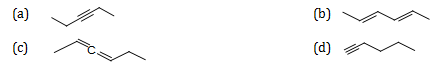Solution:

Given: molecular formula of organic compound (x) = C6H10 i.e. CnH2n–2

So, terminal alkyne reacts with HgSO4 and dil. H2SO4

Produce ketone with gives Iodoform test.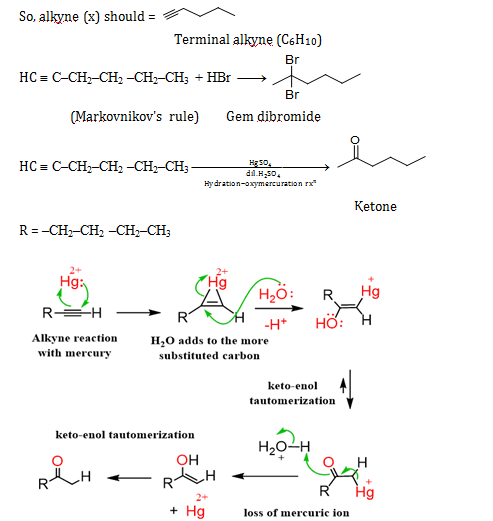Therefore, Iodoform test is shown by those groups which contain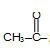group in their structure. So, option (d) satisfies all the condition.

### KVPY SA 2019 Chemistry Paper with Solutions